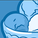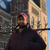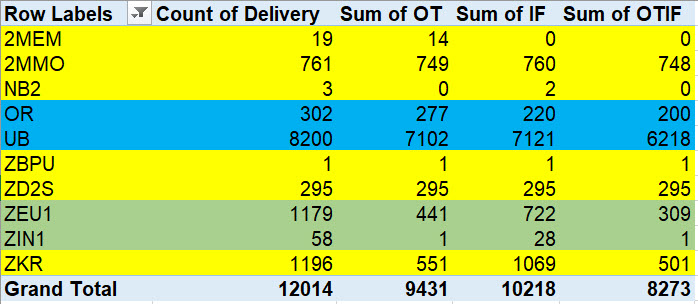# QlikView App Dev

Discussion Board for collaboration related to QlikView App Development.

Announcements
Join us at the Cloud Data and Analytics Tour! REGISTER TODAY
cancel
Showing results for
Search instead for
Did you mean:Creator III

## Pivot table help

Hey guys,

I am making a pivot table showing On Time In Full...

I have gotten to where I need to get the sum of In Full.

for my pivot table, this is my expression for In Full:

if((sum([Delivery quantity_OTIF]) - sum([Order Quantity_OTIF])) >= 0, 1, 0)

Now I am trying to get the sum of all of that data.

I am trying with this equation:

Sum(if((sum([Delivery quantity_OTIF]) - sum([Order Quantity_OTIF])) >= 0, 1, 0))

but I keep getting an error saying "Nested aggregation not allowed"

Any tips or help?

I'm just a little confused I guess because this equation DID work:

sum(if((num(date([Ac GI date_OTIF]))-num(Date([Pl GI date_OTIF]))) <= 0,1,0))

1 Solution

Accepted SolutionsSpecialist II

LOAD

Material_OTIF,

Batch_OTIF,

"Delivery quantity_OTIF",

"Order Quantity_OTIF",

"Delivery quantity_OTIF" - "Order Quantity_OTIF" as "Qty IF",

Delivery_OTIF,

"Pl GI date_OTIF",

"Ac GI date_OTIF",

SU_OTIF,

"Sales Doc_OTIF",

SaTy_OTIF

FROM [lib://DataFile/OTIF Data.xlsx]

(ooxml, embedded labels, table is [RAW DATA]);

Expression

=Sum(If([Qty IF]>=0,1,0))30 RepliesChampion

Hi,

the second equation work because date() is not an aggregation function, it is a scalar function

Read this: Aggregations and Function Classes

To make it works, you should add the Aggr() function between the two Sums.

like this:

Sum(...)

Sum(Aggr(Sum(...),...))

Sum(Aggr(Sum(Aggr(Sum(...),...)),...))

Sum(Aggr(Sum(Aggr(Sum(Aggr(Sum(...),...)),...)),...))

Hope it helps

YBCreator III
Author

I tried this, but it doesn't actually show the sum.

Sum(Aggr((sum([Delivery quantity_OTIF]) - sum([Order Quantity_OTIF])) >= 0, 1, 0))Partner

Try to add the dimensions to the Aggr list .

Please provide a sample qvw file with the expected output.Creator III
Author

Thanks for trying to help, here is the files.

If you are able to do this, the results will vary because excel only pulls the 1st 1000 rows from the data.Specialist II

Are you expecting following?Formula

=Aggr(if(sum([Delivery quantity_OTIF]) - sum([Order Quantity_OTIF]) >= 0, 1, 0), SaTy_OTIF)Creator III
AuthorSomewhere around these results. At least this much. The results from Qlik may be a little higher.Specialist II

LOAD

Material_OTIF,

Batch_OTIF,

"Delivery quantity_OTIF",

"Order Quantity_OTIF",

"Delivery quantity_OTIF" - "Order Quantity_OTIF" as "Qty IF",

Delivery_OTIF,

"Pl GI date_OTIF",

"Ac GI date_OTIF",

SU_OTIF,

"Sales Doc_OTIF",

SaTy_OTIF

FROM [lib://DataFile/OTIF Data.xlsx]

(ooxml, embedded labels, table is [RAW DATA]);

Expression

=Sum(If([Qty IF]>=0,1,0))Creator III
Author

how did I not think of this....

Thank you so much!Creator III
Author

I am trying to do the Sum of OTIF now and getting the same message. How would you go about this?

sum(if((

(sum(if((num(date([Ac GI date_OTIF]))-num(Date([Pl GI date_OTIF]))) <= 0,1,0)))

and

(Sum(If([Qty IF]>=0,1,0))) ),

1,0))